# Thin Walled Tubes Civil Engineering (CE) Notes | EduRev

## Civil Engineering (CE) : Thin Walled Tubes Civil Engineering (CE) Notes | EduRev

The document Thin Walled Tubes Civil Engineering (CE) Notes | EduRev is a part of the Civil Engineering (CE) Course Advanced Solid Mechanics - Notes, Videos, MCQs & PPTs.
All you need of Civil Engineering (CE) at this link: Civil Engineering (CE)

Thin walled tubes

In this section, we seek approximate solution to the case when, the bar, in the form of a thin walled tube is subjected to end torsion. We do not assume that the cross section of the tube to be of any particular shape. While we assume that the thickness of the cross section, t is small, we do not assume that it is uniform. Let the boundary of the cross section be defined by a function, f(x, y) = 0, the set of points constituting this boundary be denoted by ∂Ao, and the enclosed area by Ao. The boundary in the interior of the cross section is defined by the function g1(x, y) = 0, the set of points constituting this boundary is denoted by ∂Ai and the area enclosed by, Ai . Then the area of the cross section is Acs = Ao − Ai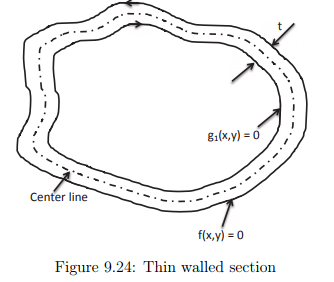In order to satisfy the requirement that the lateral surface is taction free, boundary conditions (9.107) and (9.108), the stress function has to be zero at the outer surface (9.117) and should be a constant at the inner surface (9.118). Hence, we assume that the stress function varies linearly through the thickness of the section. This assumption would be reasonable given that the thickness of the section is small. Thus,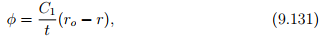where C1 is a constant, the value of φ at the inner surface, ro is the radial distance of the outer boundary from the centroid of the cross section, r is the radial distance of any point in the cross section. If ri is the radial distance of the inner boundary from the centroid of the cross section, then t = ro − ri . For the warping displacement, ψ to be single valued in the thin walled tube shown in figure 9.24,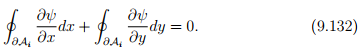Substituting equations (9.45) and (9.46) in the above equation,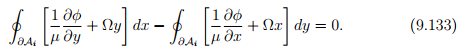Using Green’s theorem (2.271) we find that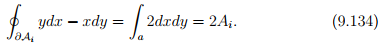Since, the stress function is assumed to vary linearly over the domain, grad(φ) = −C1/ter, where er is a unit vector normal to the boundary of the cross section. Consequently,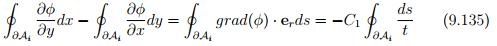Substituting equations (9.134) and (9.135) in (9.133) we obtain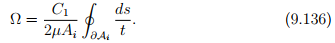Since, φ varies linearly through the thickness of the cross section, using trapezoidal rule for integration we find that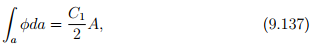where A is the area of the cross section. Here it should be pointed out that trapezoidal rule for integration gives the exact value when the variation is linear. Now, substituting equation (9.137) in (9.126) we obtain the torque to be,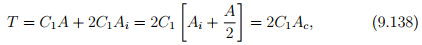where Ac is the area enclosed by the centerline of the cross section. As a consequence of the section being thin walled, Ai ≈ Ac. Therefore, from equations (9.136) and (9.138) we obtain,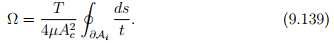Here it should be recollected that the thickness of the section can vary along the circumference of the cross section. Finally, we estimate the shear stress in the cross section. Since, the shear stress in the cross section is equal to the magnitude of grad(φ), we obtain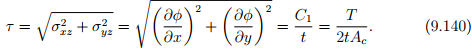Thus, τ t is constant throughout the section.

Offer running on EduRev: Apply code STAYHOME200 to get INR 200 off on our premium plan EduRev Infinity!

## Advanced Solid Mechanics - Notes, Videos, MCQs & PPTs

42 videos|61 docs

,

,

,

,

,

,

,

,

,

,

,

,

,

,

,

,

,

,

,

,

,

;